# Difference between revisions of "Cole equation of state"

The Cole equation of state  is the adiabatic version of the stiffened equation of state. (See Derivation, below.) It has the form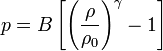$p = B \left[ \left( \frac{\rho}{\rho_0} \right)^\gamma -1 \right]$

In it,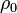$\rho_0$ is a reference density around which the density varies,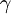$\gamma$ is the adiabatic index, and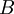$B$ is a pressure parameter.

Usually, the equation is used to model a nearly incompressible system. In this case, the exponent is often set to a value of 7, and$B$ is large, in the following sense. The fluctuations of the density are related to the speed of sound as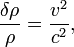$\frac{\delta \rho}{\rho} = \frac{v^2}{c^2} ,$

where$v$ is the largest velocity, and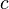$c$ is the speed of sound (the ratio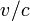$v/c$ is Mach's number). The speed of sound can be seen to be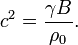$c^2 = \frac{\gamma B}{\rho_0}.$

Therefore, if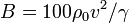$B=100 \rho_0 v^2 / \gamma$, the relative density fluctuations will be about 0.01.

If the fluctuations in the density are indeed small, the equation of state may be approximated by the simpler: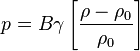$p = B \gamma \left[ \frac{\rho-\rho_0}{\rho_0} \right]$

It is quite common that the name "Tait equation of state" is improperly used for this EOS. This perhaps stems for the classic text by Cole calling this equation a "modified Tait equation" (p. 39).

## Derivation

Let us write the stiffened EOS as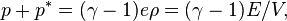$p+ p^* = (\gamma -1) e \rho = (\gamma -1) E / V ,$

where E is the internal energy. In an adiabatic process, the work is the only responsible of a change in internal energy. Hence the first law reads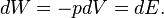$dW= -p dV = dE .$

Taking differences on theEOS,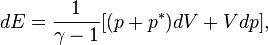$dE = \frac{1}{\gamma-1} [(p+p^*) dV + V dp ] ,$

so that the first law can be simplified to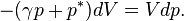$- (\gamma p + p^*) dV = V dp.$

This equation can be solved in the standard way, with the result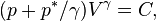$( p + p^* / \gamma) V^\gamma = C ,$

where C is a constant of integration. This derivation closely follows the standard derivation of the adiabatic law of an ideal gas, and it reduces to it if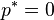$p^* =0$.

If the values of the thermodynamic variables are known at some reference state, we may write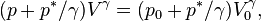$( p + p^* / \gamma) V^\gamma = ( p_0 + p^* / \gamma) V_0^\gamma ,$

which can be written as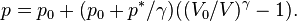$p = p_0 + ( p_0 + p^* / \gamma) ( (V_0/V)^\gamma - 1 ) .$

Going back to densities, instead of volumes,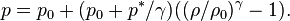$p = p_0 + ( p_0 + p^* / \gamma) ( (\rho/\rho_0)^\gamma - 1) .$

Comparing with the Cole EOS, we can readily identify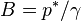$B = p^* / \gamma$

Moreover, the Cole EOS differs slightly, as it should read (as indeed does in e.g. the book by Courant)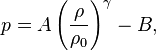$p = A \left( \frac{\rho}{\rho_0} \right)^\gamma - B ,$

with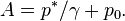$A = p^* / \gamma + p_0 .$

This difference is negligible for liquids but for an ideal gas$p^*=0$ and there is a huge difference, B being zero and A being equal to the reference pressure.

Now, the speed of sound is given by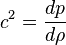$c^2=\frac{dp}{d\rho}$

with the derivative taken along an adiabatic line. This is precisely our case, and we readily obtain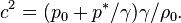$c^2= ( p_0 + p^* / \gamma) \gamma /\rho_0 .$

From this expression a value of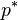$p^*$ can be deduced. For water,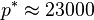$p^*\approx 23000$ bar, from which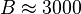$B\approx 3000$ bar. If the speed of sound is used in the EOS one obtains the rather elegant expression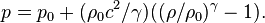$p = p_0 + ( \rho_0 c^2 / \gamma) ( (\rho/\rho_0)^\gamma - 1) .$